# Python range() Function

This article deals with one of the most widely used built-in function of Python, that is range() function. You'll learn all about range() function, step by step in this tutorial. This tutorial deals with:

• What is range() Function
• Syntax of range() Function
• Example Programs of range() Function
• range() Function with Two Arguments
• range() Function with Three Arguments
• range() Function to generate sequence in Reverse
• Generate sequence using range() based on user's requirement

Don't skip this tutorial, until getting complete understanding on range(), as it plays like a trump card in most of the program created using loops

## What is range() Function ?

As name suggests, range() creates/generates a sequence of numbers. That is, using range(), we can generate some set of numbers. The order of sequence is defined through its argument(s) passed.

Most of the time, range() used in for loop. Since for loop is used to execute some set of statements, some required number of times. And range() helps to do that type of task.

## range() Function Syntax

The syntax of using range() function is:

`range(start, stop, increment_by)`

The first (start) and third (increment_by) arguments are optional. Let's understand about all the three arguments in detail.

Here start indicates the number/value from where the generation of sequence of numbers gets started. And stop is the number/value that indicates the stoppage of generation of sequence of number. Finally, the third argument, that is increment_by is the value that is used to increment the sequence of numbers by this value.

Keep in Mind - start is included, whereas stop is excluded. For example, range(10, 15) gives 10, 12, 13, 14. Don't worry, you'll get to know about its working later on, in this tutorial.

In all the three arguments, the second one, that is stop is necessary. That is:

• If there is only one argument passed to range(), then it gets treated as stop
• If there are two arguments passed to range(), then the first argument gets treated as start, whereas the second argument indicates to stop
• And if there are three arguments passed to range(), then first is for start, second is for stop, and third argument is the value that is used to increment the sequence by its value, every time

If you're not getting an idea, on what I'm trying to say, then just read it and continue. Because after looking at some of the examples given below, I'm sure, you'll get to know everything about the topic.

Important - The default value of start is 0, whereas increment_by is 1. That is, if you do not provide these two arguments to range(). Or if you provide only one argument to it, then that one argument is for stop. But start automatically gets initialized with 0, where as increment_by automatically gets initialized with 1.

Note: All of the three arguments must be an integer. That is, don't pass any argument as real numbers like 1.4, 5.2 etc.

## range() Function Example

Since programming is the thing, that can not be learn by theory only, as it must requires practical implementation of the code given in theory part. Therefore, I've provided almost all the programs that uses total variety of range() function. Let's start with very basic one:

```nums = range(10)
for n in nums:
print(n)```

The output produced by above program is: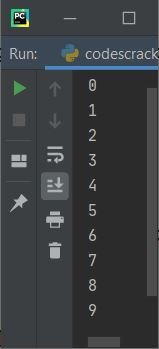In above program, the statement:

`nums = range(10)`

generates a sequence of numbers, that are from 0 to 10, where 0 is included but 10 is not. Since I've not provided rest of the two arguments. Therefore, the start gets automatically initialized with 0, whereas increment_by initialized with 1. That is, the generation of sequence of numbers, gets started with 0, and increment by 1 each time. This process continues until 1 less than the number (stop's value).

Now let's change the above program with the program given below:

```nums = range(10)
print(nums)```

With this program, you'll get following output: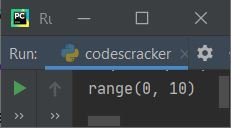That shows, if you iterate nums, then you will get a sequence of numbers starting with 0, and ends with 9 (included), like shown in the previous program's sample output. You can also directly use range() function (in for loop) like shown in the program given below:

```for n in range(10):
print(n)```

You'll get the same output, that is, a sequence of numbers from 0 to 9. You can also create list using range() function, like shown in the program given below:

```nums = list(range(10))
print(nums)```

Now the output produced by above program is: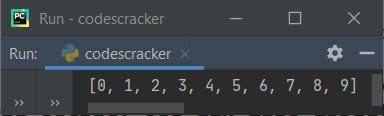The same program can also be replaced with:

```nums = range(10)
nums = list(nums)
print(nums)```

The output will be same as of previous program.

### range() Function with Two Arguments

Since all the above example programs of range() function is based on only argument. Therefore let's start with providing more than one arguments like shown in the very basic example given below:

```for n in range(1, 10):
print(n)```

Because I've provided two arguments to range(). Then first argument automatically gets treated as start's value, whereas the second argument automatically gets treated as stop's value. Therefore the above program produces the output like shown in the snapshot given below:As you can see from above sample output, the program produces a sequence of number, that is from 1 to 10, not 0 to 9. Because I've provided 1 as the number to start the sequence with.

### range() Function with all Three Arguments

Now let's use all the three arguments:

```for n in range(0, 50, 5):
print(n)```

The above program produces the following output: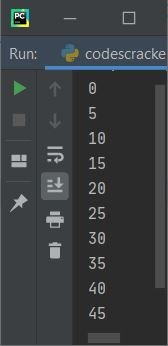As you can see, the third argument is 5, therefore each time the sequence gets incremented by 5.

### range() Function to Generate Sequence in Reverse

The range() function can also be used to generate/create sequence of numbers in reverse. To do this task, these are the two basic things, that must be followed while doing this task:

• The first argument must be greater than second
• The third argument must be with minus (-) sign

Let's take a very basic example, that generates sequence of numbers using range() in reverse, of course:

```nums = range(10, 0, -1)
print(list(nums))```

The range(10, 0, -1) indicates that the sequence gets started with 10, decremented by 1 (because I've used -1), and continues generating the sequence of numbers before 0. Here is the output produced by above program: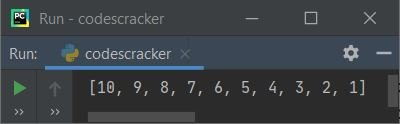Here is another program:

```nums = range(100, 0, -10)
print(list(nums))```

This will generate/prints [100, 90, 80, 70, 60, 50, 40, 30, 20, 10].

Note: Always use minus (-) for the third argument. That is increment_by must gets replaced with a value along with minus (-).

### Generate Sequence of Numbers using range() Based on User's Requirement

This is the master program for you, that helps you to understand about range() function completely. Let's have a look at the example program and its sample run given below:

```print("Enter the value to start with: ")
start_value = int(input())
print("Enter the value to end before: ")
stop_value = int(input())
print("Enter the value to increment by, each time: ")
increment_by_value = int(input())

print("\nGenerating sequence of numbers, based on your requirement:")
for n in range(start_value, stop_value, increment_by_value):
print(n)```

Here is its sample run with user inputs 24 (as value to start with), 37 (as value to end before), and 3 (as value to increment by):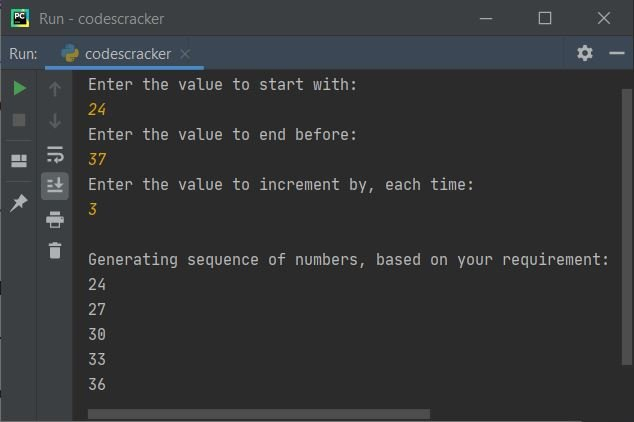Here is another sample run with user inputs, 37, 24, and -3: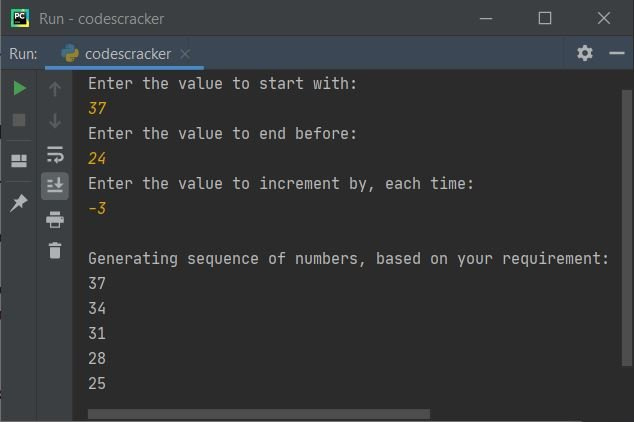Now I think, you've got the complete understanding about range() function in Python. That is very good, as it is going to use a lot of times while you program in Python.

Python Online Test

« Previous Function Next Function »